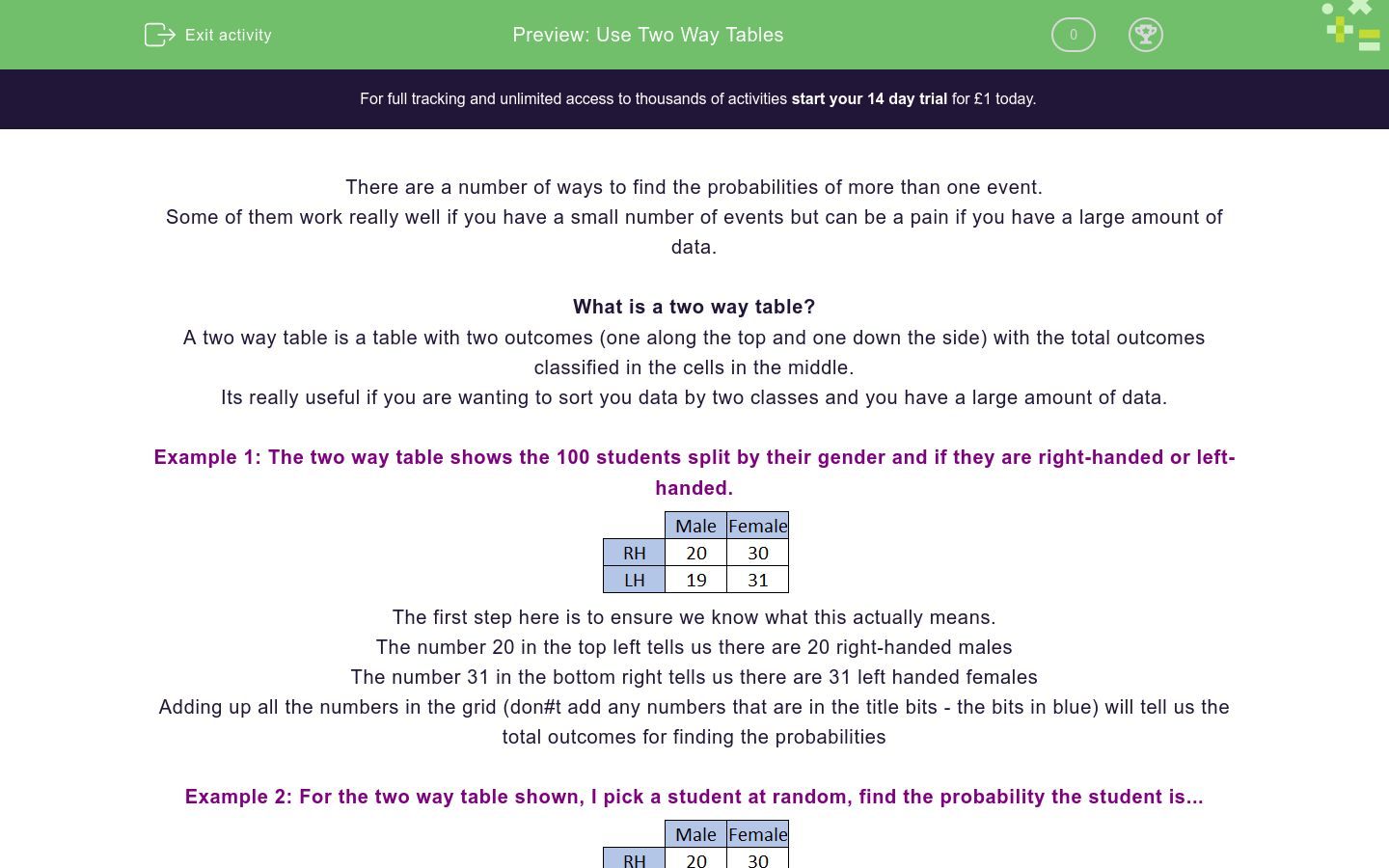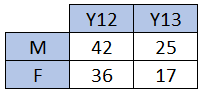# Use Two Way Tables

In this worksheet, students practise using two-way tables to find probabilities.Key stage:  KS 4

GCSE Subjects:   Maths

GCSE Boards:   AQA, Eduqas, Pearson Edexcel, OCR

Curriculum topic:   Probability

Curriculum subtopic:   Probability, Combined Events and Probability Diagrams

Difficulty level:### QUESTION 1 of 10

There are a number of ways to find the probabilities of more than one event.

Some of them work really well if you have a small number of events but can be a pain if you have a large amount of data.

What is a two way table?

A two way table is a table with two outcomes (one along the top and one down the side) with the total outcomes classified in the cells in the middle.

Its really useful if you are wanting to sort you data by two classes and you have a large amount of data.

Example 1: The two way table shows the 100 students split by their gender and if they are right-handed or left-handed.The first step here is to ensure we know what this actually means.

The number 20 in the top left tells us there are 20 right-handed males

The number 31 in the bottom right tells us there are 31 left handed females

Adding up all the numbers in the grid (don#t add any numbers that are in the title bits - the bits in blue) will tell us the total outcomes for finding the probabilities

Example 2: For the two way table shown, I pick a student at random, find the probability the student is...a) A right handed male

There are 20 right handed males and 100 people in total.

This is a probability of 20/100 which cancels to 1/5

b) Female

There are 61 females in this and 100 people in total

This is a probability of 61/100

c) A left-handed girl

There are 31 left handed girls out of the 100 in total.

This is a probability of 31/100

Sample space diagrams are used to list the groups of...

A two-way table shows the gender and year groups in a sixth form college.If I select a student at random, find the probability the student is in year 13.

A two-way table shows the gender and year groups in a sixth form college.If I select a student from year 12, what is the probability I get a girl?

A two-way table shows the gender and year groups in a sixth form college.Match the given situation with its probability.

## Column B

Probabililty I pick a boy
71/120
Probability I pick a boy in year 12
7/120
Probability I pick a girl
53/120
Given I pick a boy, the chance he is in year 12
42/67

A Vet surveys all the customers that come into his surgery one week and puts the information into a two way table.If I select a random customer, what would the chance be that they are a man who owns a dog?

A Vet surveys all the customers that come into his surgery one week and puts the information into a two way table.If I selected a woman, what would be the chance she owned a cat?

A Vet surveys all the customers that come into his surgery one week and puts the information into a two way table.Match the given situation with its probability.

## Column B

Probabililty I pick a cat
7/20
Probability I pick a man
37/80
Probability I pick a woman with a dog
7/16
Given I choose a dog the chance the owner is femal...
28/43

A school class survey all the cars in a car park and record the results in a two way table.If I picked a random car, what is the probability it would be a black car?

A school class survey all the cars in a car park and record the results in a two way table.If I picked a car from the vauxhalls, what is the probability it would be blue?

A school class survey all the cars in a car park and record the results in a two way table.Match the given situation with its probability.

## Column B

Probabililty I pick a nissan
6/23
Probability I pick a blue car
23/50
Probability I pick a black vauxhall
13/50
Given I choose a blue car the chance it is a toyot...
1/20
• Question 1

Sample space diagrams are used to list the groups of...

EDDIE SAYS
Sample space diagrams are useful when we sort a large amount of data into groups of outcomes.
• Question 2

A two-way table shows the gender and year groups in a sixth form college.If I select a student at random, find the probability the student is in year 13.

7/20
EDDIE SAYS
The table tells us that there are 25 boys and 17 girls in year 13. Adding all the numbers together gives 120 students. The probability I get a year 3 student is therefore 42/120 which can cancel to ...
• Question 3

A two-way table shows the gender and year groups in a sixth form college.If I select a student from year 12, what is the probability I get a girl?

6/13
EDDIE SAYS
You might have been caught out with this one. The first part of the question says' If I select a student from year 12,' This limits the total outcomes to the students in year 12 (36 + 42 = 78) Of these 36 are girls so my probability is 36/78 which cancels to...
• Question 4

A two-way table shows the gender and year groups in a sixth form college.Match the given situation with its probability.

## Column B

Probabililty I pick a boy
71/120
Probability I pick a boy in year ...
7/120
Probability I pick a girl
53/120
Given I pick a boy, the chance he...
42/67
EDDIE SAYS
Remember with each of these questions to ask... 1) How many is it out of? 2) How many satisfy the condition? Once you have these, you can create the probability and try to cancel it down.
• Question 5

A Vet surveys all the customers that come into his surgery one week and puts the information into a two way table.If I select a random customer, what would the chance be that they are a man who owns a dog?

3/16
EDDIE SAYS
It's very simple to see that there are 15 men who own dogs. We also need to find out how many people in total came in. If we add up all the numbers in the two-way table, we find there were 80 visitors. If we put these together we get 15/80 which cancels to...
• Question 6

A Vet surveys all the customers that come into his surgery one week and puts the information into a two way table.If I selected a woman, what would be the chance she owned a cat?

17/45
EDDIE SAYS
We can see straight away that there are 17 women who own cats. Did you spot the questions said 'If you select a woman'? This means we are only picking from the women which means our probability is out of 45
• Question 7

A Vet surveys all the customers that come into his surgery one week and puts the information into a two way table.Match the given situation with its probability.

## Column B

Probabililty I pick a cat
37/80
Probability I pick a man
7/16
Probability I pick a woman with a...
7/20
Given I choose a dog the chance t...
28/43
EDDIE SAYS
Remember with each of these questions to ask... 1) How many is it out of? 2) How many satisfy the condition? Once you have these, you can create the probability and try to cancel it down.
• Question 8

A school class survey all the cars in a car park and record the results in a two way table.If I picked a random car, what is the probability it would be a black car?

27/100
EDDIE SAYS
We need to find two pieces of information for this. 1) The number of black cars - 10 + 23 + 21 = 58 2) The total number of cars - Add up all the numbers to get 200 This gives a probability of 58/200 which can be cancelled to...
• Question 9

A school class survey all the cars in a car park and record the results in a two way table.If I picked a car from the vauxhalls, what is the probability it would be blue?

17/52
EDDIE SAYS
We need to find two pieces of information for this. 1) The number of vauxhall cars - 52 2) The total number of blue vauxhall cars - 17
• Question 10

A school class survey all the cars in a car park and record the results in a two way table.Match the given situation with its probability.

## Column B

Probabililty I pick a nissan
13/50
Probability I pick a blue car
23/50
Probability I pick a black vauxha...
1/20
Given I choose a blue car the cha...
6/23
EDDIE SAYS
Remember with each of these questions to ask... 1) How many is it out of? 2) How many satisfy the condition? Once you have these, you can create the probability and try to cancel it down.
---- OR ----

Sign up for a £1 trial so you can track and measure your child's progress on this activity.

### What is EdPlace?

We're your National Curriculum aligned online education content provider helping each child succeed in English, maths and science from year 1 to GCSE. With an EdPlace account you’ll be able to track and measure progress, helping each child achieve their best. We build confidence and attainment by personalising each child’s learning at a level that suits them.

Get started